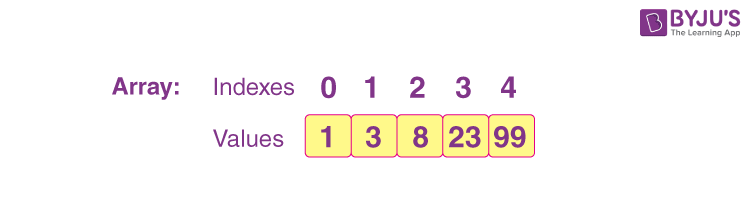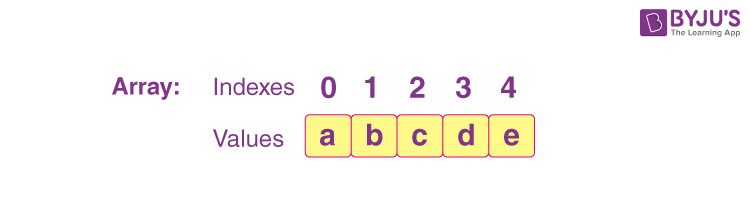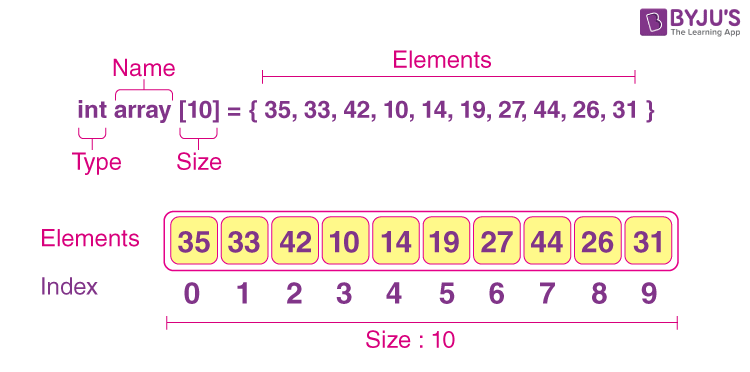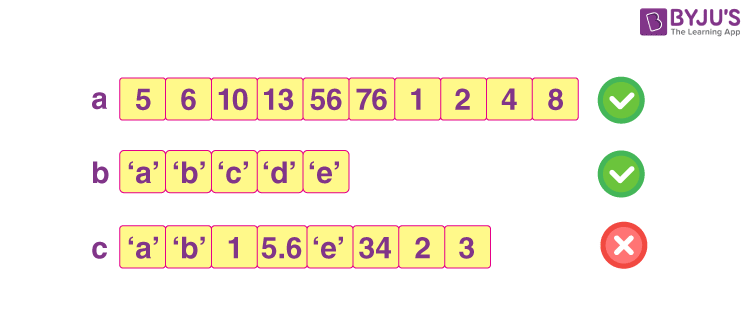# Introduction To Array - Notes For GATE

An array is an important topic belonging to the computer science family. And, when it comes to a competitive examination like GATE, you have to deep dive into each perspective of the array. In this article, we have covered all the topics relevant to the array. We hope the notes for the CSE topics will help you understand this topic in a better way.

Topic of Contents

## What is Array?

An array is a group of similar elements or data items of the same type collected at contiguous memory locations. In simple words, we can say that in computer programming, arrays are generally used to organize the same type of data.

Array for Integral value:Array for Character value:## Representation of an Array:

Arrays can be represented in several ways, depending on the different languages. To make you understand, we can take one example of the C language. The picture below shows the representation of the array.Arrays always store the same type of values. In the above example:

• int is a type of data value.
• Data items stored in an array are known as elements.
• The location or placing of each element has an index value.

Important: Array can store only the same type of data items. From the below example you can see how it works:• In the array a, we have stored all integral values (same type)
• In the array b, we have stored all char values (same type)
• In the array c, there is integral, float, char all types of values and this is not something an array can store so, option 3 is wrong because an array cannot store different types of values.

## Declaration Syntax of Array:

VariableType VariableName[Sequence of Elements];

Example 1: For integral value

int A;

Here 10 means, this array A can have 10 integer elements.

 2 5 8 44 21 11 7 9 3 1

Example 2: For character value

char B;

This array B can have 10 character elements.

 f d a b n j l s e y

## Initialization of an Array:

If an array is described inside a function, the elements will have garbage value. And in case an array is static or global, its elements will be initialized automatically to 0.

We can say that we can simply initialize elements of an array at the time of declaration and for that, we have to use the proper syntax:

Syntax: datatype Array_Name[size] = { value1, value2, value3, ….. valueN };

## Types of Arrays:

There are two types of arrays:

• One-Dimensional Arrays
• Multi-Dimensional Arrays

## One -Dimensional Arrays

A one-dimensional array is a kind of linear array. It involves single sub-scripting. The [] (brackets) is used for the subscript of the array and to declare and access the elements from the array.

Syntax: DataType ArrayName [size];

For example: int a;

## Multi-Dimensional Arrays

In multi-dimensional arrays, we have two categories:

• Two-Dimensional Arrays
• Three-Dimensional Arrays
1. 1. Two-Dimensional Arrays

An array involving two subscripts [] [] is known as a two-dimensional array. They are also known as the array of the array. Two-dimensional arrays are divided into rows and columns and are able to handle the data of the table.

Syntax: DataType ArrayName[row_size][column_size];

For Example: int arr;

2. Three-Dimensional Arrays

When we require to create two or more tables of the elements to declare the array elements, then in such a situation we use three-dimensional arrays.

Syntax: DataType ArrayName[size1][size2][size3];

For Example: int a;

Visit and explore, Difference Between One-Dimensional and Two-Dimensional Array

• It is a better version of storing the data of the same size and same type.
• It enables us to collect the number of elements in it.
• Arrays have a safer cache positioning that improves performance.
• Arrays can represent multiple data items of the same type using a single name.

• In an array, it is essential to identify the number of elements to be stored.
• It is a static structure. It means that in an array, the memory size is fixed.
• When it comes to insertion and deletion, it is a bit difficult because the elements are stored sequentially and the shifting operation is expensive.

## Video on Arrays## Practice Problem – Array

Q. A program P reads in 500 integers in the range [0, 100] representing the cores of 500 students. It then prints the frequency of each score above 50. What would be the best way for P to store the frequencies?

1. An array of 50 numbers
2. An array of 100 numbers
3. An array of 500 numbers
4. A dynamically allocated array of 550 numbers

Q. The following C functions in which size is the number of elements in the array E:

int MyX(int *E, unsigned int size){

int Y = 0;

int Z;

int i,j,k;

for(i = 0; i < size; i++)

Y = Y + E[i];

for(i = 0; i < size; i++)

for(j = i; j < size; j++){

Z = 0;

for(k = i; k <= j; k++)

Z = Z + E[k];

if(Z > Y)

Y = X;

}

return Y;

}

The value returned by the function MyX is the

1. Maximum possible sum of elements in any sub-array of array E.
2. Maximum element in any sub-array of array E.
3. Sum of the maximum elements in all possible sub-arrays of array E.
4. The sum of all elements in the array E.

Q1

### What is an array answer?

An array is a data structure, which can store a fixed-size collection of elements of the same data type.

Q2

### Types of arrays?

There are two types of array:

• Two-dimensional array.
• Multi-dimensional array
Q3

### How to represent two-dimensional arrays?

Syntax: DataType ArrayName[row_size][column_size];
For Example int arr;

Keep learning and stay tuned to get the latest updates on GATE Exam along with GATE Eligibility Criteria, GATE 2023, GATE Admit Card, GATE Syllabus for CSE (Computer Science Engineering), GATE CSE Notes, GATE CSE Question Paper, and more.

Also Explore,